Notes taken from watching 3Blue1Brown series: Nerual networks.

• But what is a nerual network?
• Back Propagation

## Chapter 1: Overview

Consider Nerual network:

• What are the neurons?
• Functions, which take several numbers as input and give a number as output
• How are they linked together?

Layers (Each layer made up of neurons):

• The activations of one layer determines the activations of the next layer.

What those middle layers might be doing?

• It may be holding subcomponents of the image.
• Input layer -> Edge layer -> Pattern layer -> Output Digit Layer (?)

Edge Detection Example

• Assign a weight to each connection between the neuron and the neurons from the former layer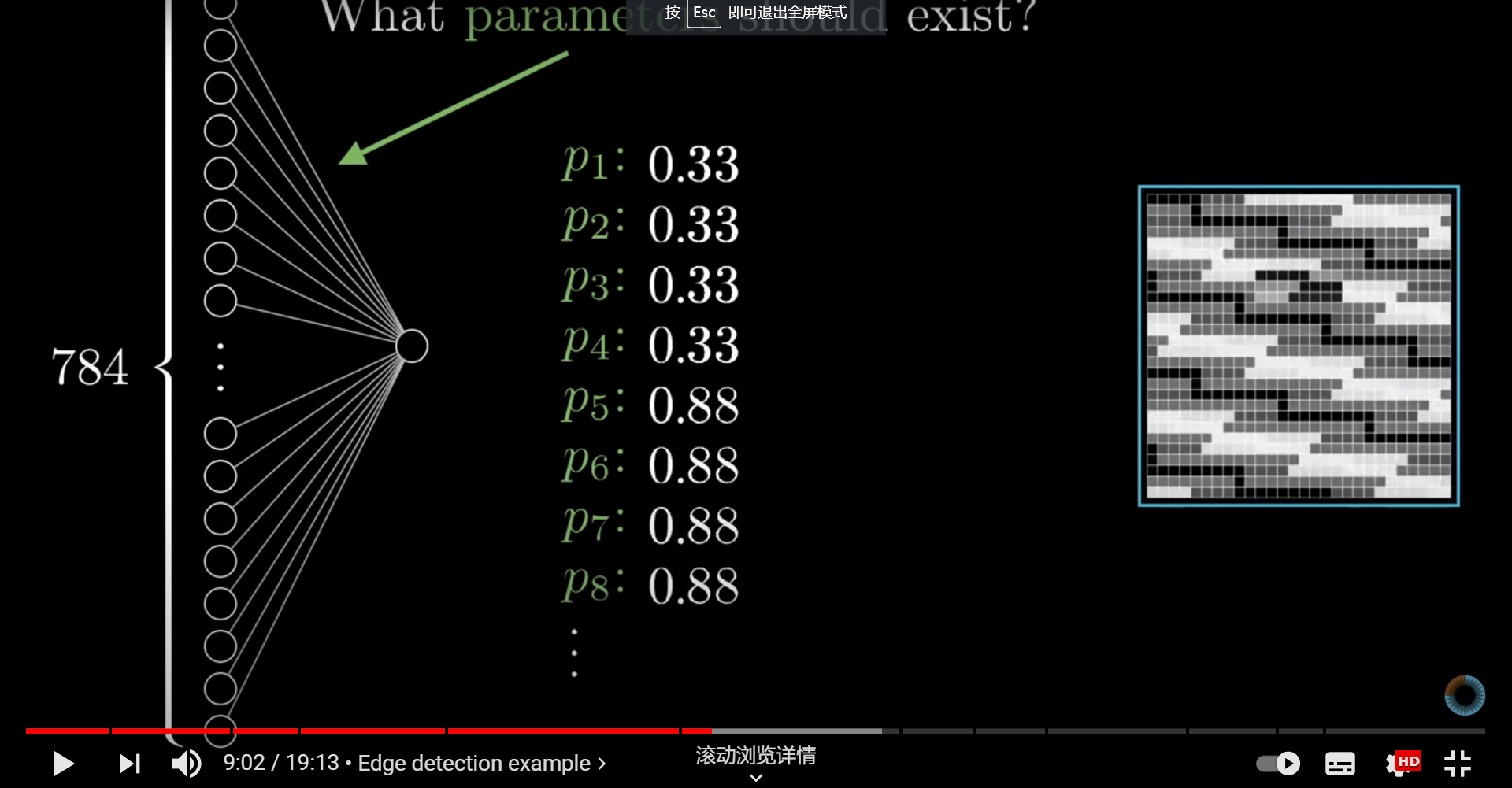• Let activations from the last layer be $a_1, a_2, a_3, …a_n$​ and the weight numbers be $w_1, w_2, w_3, …w_n$​​.
• Let $w_1a_1 + w_2a_2 + … + w_na_n$ represent the neuron activation? No! We have to make the range of activation between [0, 1], but the result comes along with any possible real number.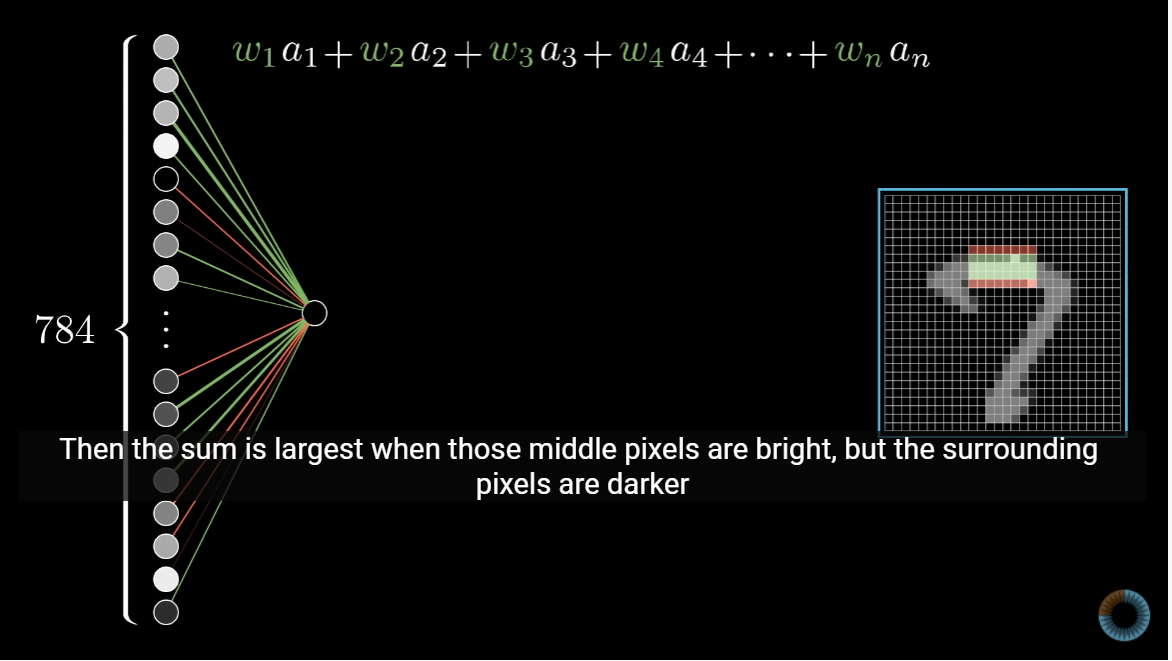• We could use the sigmoid function, or the logistic curve to solve this. $\sigma(x) = \frac 1 {1+e^{-x}}$​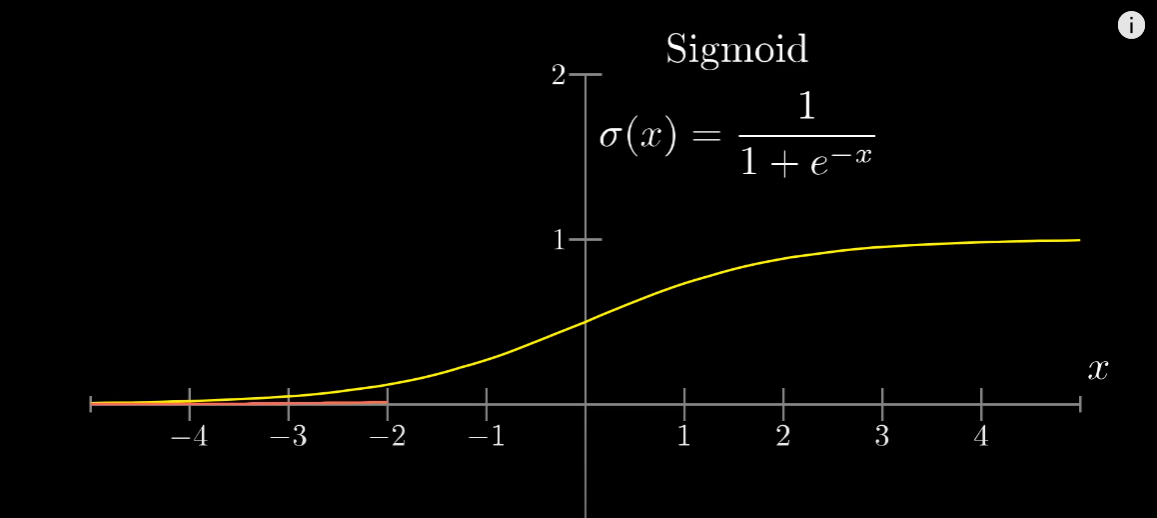• So can we let the activation of the neuron be $\sigma(w_1a_1 + w_2a_2 + … + w_na_n)$​, which is basically a measure of how positively the relevant weighted sum is?
• Well, maybe we need some bias, say, only activate when $w_1a_1 + w_2a_2 + … + w_na_n > 10$?
• So finally we get, the activation of the neuron, which is $\sigma(w_1a_1 + w_2a_2 + … + w_na_n + bias)$, which is -10 in this case.​​

Counting weights and biases

• All described above is just above one specific neuron, and in fact, in a middle layer, we have several neurons!
• Take the video example, just a two-middle-layered network have more than 13k parameters to tweak!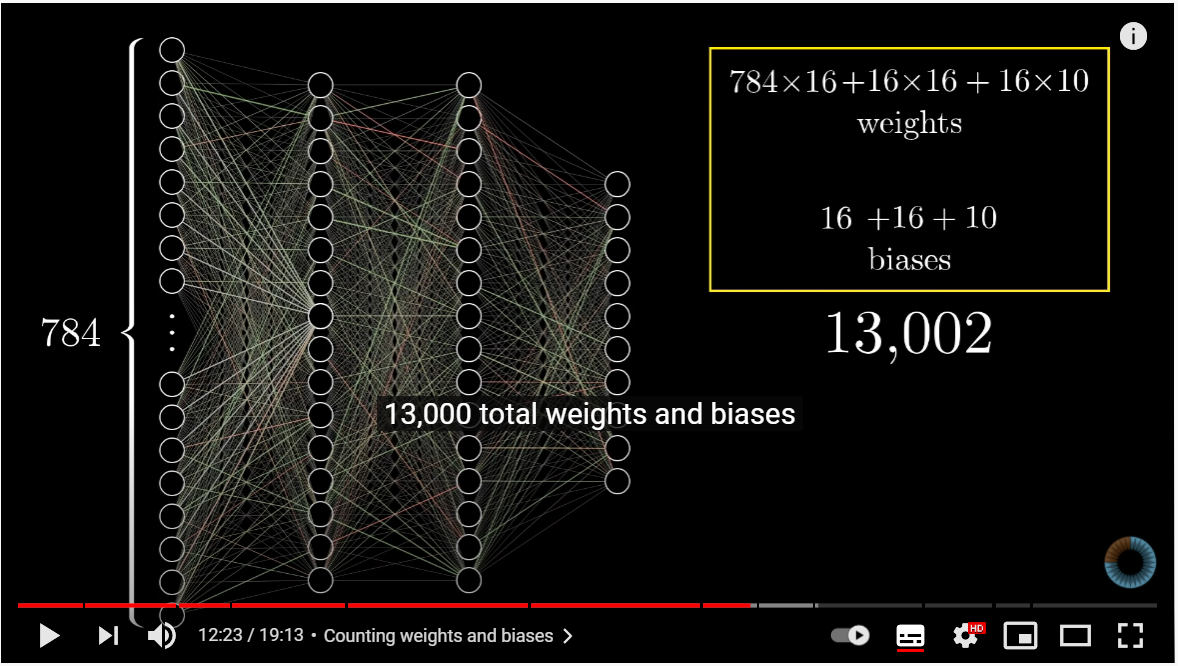• So when we talk about learning, it is about finding the right weights and biases to make the network behave in the right way.

Notations and linear algebra

• Let activations from one layer be a column vector: $\begin {bmatrix} a_1^{(0)}\\ a_2^{(0)}\\ \vdots\\ a_n^{(0)}\\ \end {bmatrix}$​​​​​​

• Let weights of connection between two adjacent layers be a matrix: $\begin {bmatrix} w_{1,1} & w_{1,2} & \cdots & w_{1,n} \\ w_{2,1} & w_{2,2} & \cdots & w_{2,n} \\ \vdots & \vdots & \ddots & \vdots \\ w_{k,1} & w_{k,2} & \cdots & w_{k,n} \end {bmatrix}$​​

• Row $i$ of the matrix represents the connection weight between neuron $i$ with the neurons from the last layer.
• Let the biases be in a column vector: $\begin {bmatrix} b_1\\ b_2\\ \vdots\\ b_n\\ \end {bmatrix}$​​​​

• And let $\sigma(\begin {bmatrix} x\\ y\\ \vdots\\ z\\ \end {bmatrix}) \ := \ \begin {bmatrix} \sigma(x)\\ \sigma(y)\\ \vdots\\ \sigma(z)\\ \end {bmatrix}$

• So we get our notation now: $a^{(2)} = \sigma(W^{(2)}a^{(1)}+b^{(2)})$​​​

• $a^{(i)}$: the activations of the $i$​-th layer
• $W^{(i)}$: connection weight matrix between layer $i$ and $i-1$
• $b^{(i)}$: biases of neurons in the layer $i$

Using training data

• We can divide our data with labels into two groups, the training group and the testing group.
• Firstly, we can use the data in the training group to train our network.
• Then we could use the test group to check its accuracy.

Cost Function

• Review: Nerual network function
• Input: 784 numbers (pixels)
• Output: 10 numbers
• Parameters: 13k weights or biases
• But the cost function might be like…
• Input: 13k weights or biases
• Output: 1 single number (namely the cost)
• Parameters: Different set of training examples
• Notation
• $C(w_1, w_2, \cdots, w_{13002}) := \frac 1 {2n} \sum_x|| y(x)-a ||^2$​
• Now we can just try to solve this problem: how to find the minimum of $C$ and the corresponding set of $w$​!
• It is hard to solve this minimum problem using mathematic methods when the amount of parameters is high, but we can…
• Start at an old input
• Figure out which direction you should step to make the cost lower
• And that direction is: $-\nabla C$​
• So we could just choose $\Delta v =-\eta\nabla C$​.
• In which $\eta$​ is a small, positive parameter (known as learning rate)
• Then we can make $v \rightarrow (v’=v-\eta\nabla C)$​ in every iteration

Anaylsing the network

• Does the network’s middle layer really doing what was imagined? Namely, edges, patterns, etc.?
• Not at all!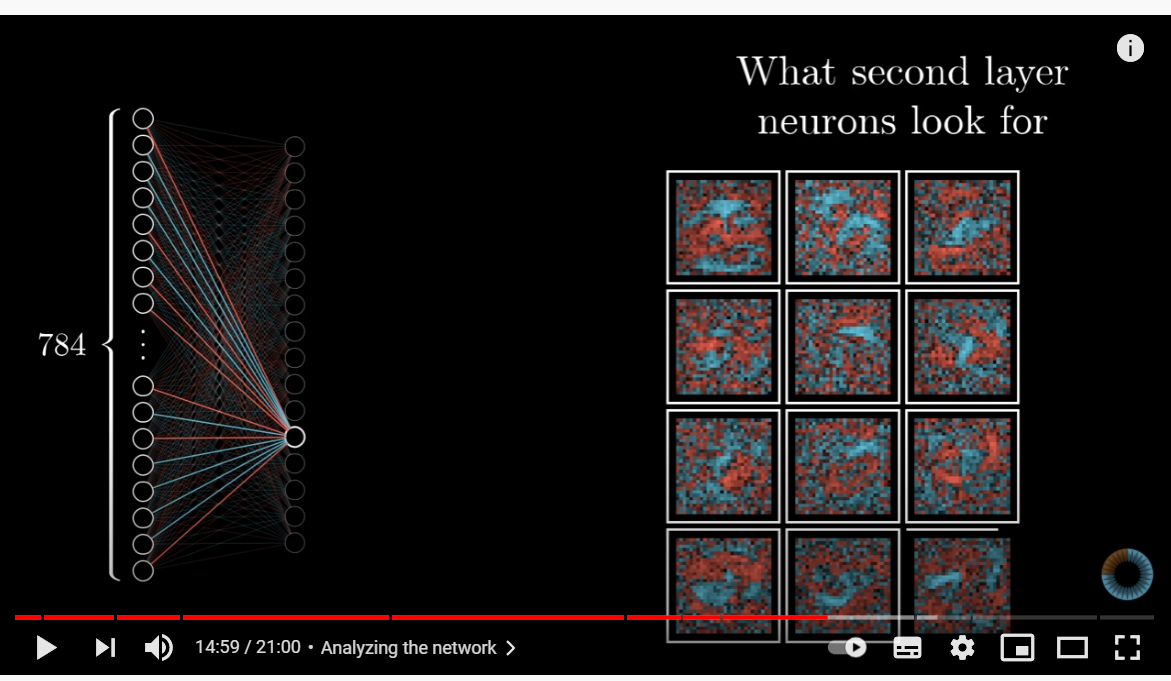• The magnitude of each element in the gradient is indicating how sensitive the cost function is to each weight or bias.

## Chapter 3: Back Propagation

What is back propagation?

• It is an algorithm for computing the gradients of the cost function.

• It takes the computer rather long time to add up the influence of every single training example.
• So we can randomly shuffle our training data, then divide it into mini-batches.
• Then we can compute a step according to the mini-batch.

Chain rule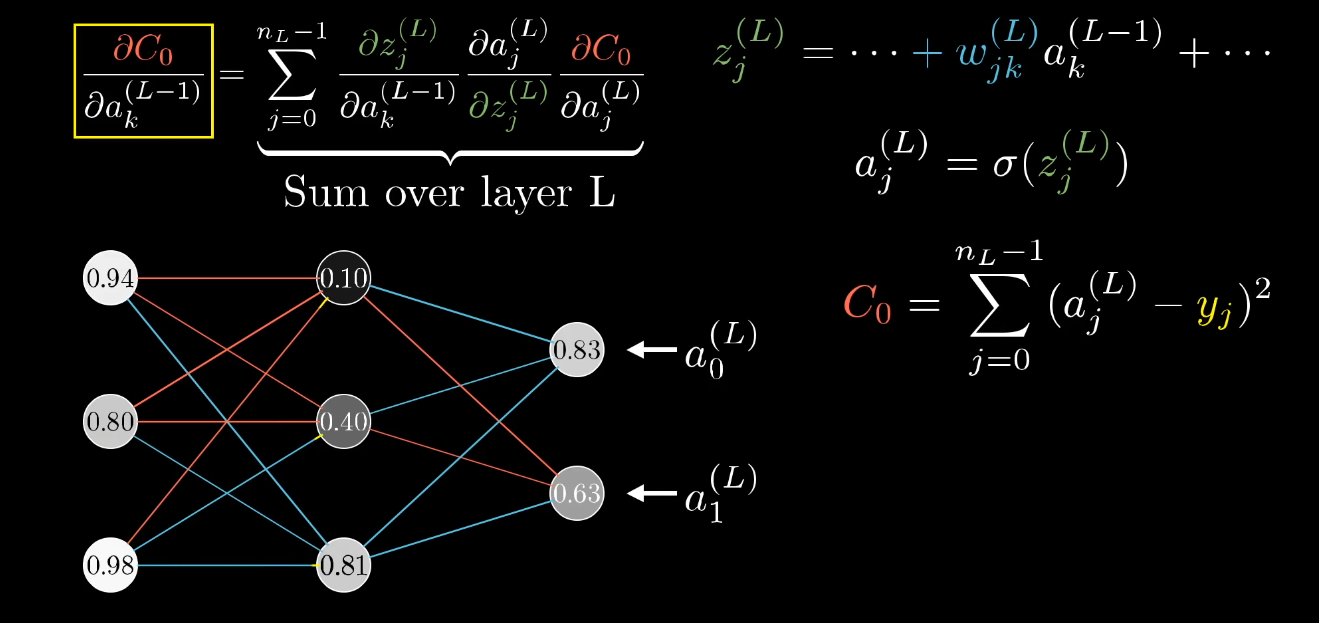graph LR
subgraph "Layer m-1"
A0("1")
A1("2")
A2("3")
A3("4")
end
subgraph "Layer m"
B2("output(i-1)")
B0("output(i)")
B1("output(i+1)")
end
subgraph "Anticipated label"
C2("y(i-1)")
C0("y(i)")
C1("y(i+1)")
end
A0 --> B0
A1 --> B0
A2 --> B0
A3 --> B0
B0 --> C0
B1 --> C1
B2 --> C2
• We remember $\begin {bmatrix} a_1^{(2)}\\ a_2^{(2)}\\ \vdots\\ a_k^{(2)}\\ \end {bmatrix}= \sigma (\begin {bmatrix} w^{(2)}_{1,1} & w^{(2)}_{1,2} & \cdots & w^{(2)}_{1,n} \\ w^{(2)}_{2,1} & w^{(2)}_{2,2} & \cdots & w^{(2)}_{2,n} \\ \vdots & \vdots & \ddots & \vdots \\ w^{(2)}_{k,1} & w^{(2)}_{k,2} & \cdots & w^{(2)}_{k,n} \end {bmatrix}\begin {bmatrix} a_1^{(1)}\\ a_2^{(1)}\\ \vdots\\ a_n^{(1)}\\ \end {bmatrix} + \begin {bmatrix} b_1^{(2)}\\ b_2^{(2)}\\ \vdots\\ b_k^{(2)}\\ \end {bmatrix})$
• Namely $a^{(L)}_i = \sigma( \sum_jw^{(L)}_{i, j}a_j^{(L-1)}+ b^{(L)})$​​​​​.​
• We denote this by $z^l = w^la^{l-1}+b^l$​ and $a^l = \sigma(z^l)$​
• And we have $C(\vec W, \vec b) = \frac 1 {2n} \sum_x|| y(x)-a ||^2$
• We assume that $C = \frac 1 n \sum_x C_x$​, in which $C_x = \frac 1 2 ||y-a^{L}||^2$​​​
graph RL
CX("$C$")
aE("$a^L$")
zE("$z^L$")
wE("$w^L$")
aEL("$a^{L-1}$")
bE("$b^L$")
zEL("$z^{L-1}$")
wEL("$w^{L-1}$")
aELL("$a^{L-2}$")
bEL("$b^{L-1}$")

aE -->|"$(y_x-a^L_x)^2$"| CX
zE -->|"$\sigma$"| aE
wE --> zE
aEL --> zE
bE --> zE
zEL -->|"$\sigma$"| aEL
wEL --> zEL
aELL --> zEL
bEL --> zEL
dot("$\cdots$") --> aELL
• Then the core equations of back propagation…
• $\frac {\partial C} {\partial z_i^L} = \frac {\partial C} {\partial a_i^L}\frac {\partial a_i^L} {\partial z_i^L} = (a_i^L-y_i)\sigma’(z_i^L)$​​​​ (Initialize)​
• That is $\delta := \frac {\partial C} {\partial z^L} = (a^L-y) \odot \sigma’(z^L)$​​
• According to $z^{(L)}_i = \sum_jw^{(L)}_{i, j}a_j^{(L-1)}+ b_i^{(L)}$​​​​, suppose we have calculated $\frac {\partial C} {\partial z^M_i}$​​​ for all neuron $i$​​​ in layer $M$​​​.
• How can we get $\frac {\partial C} {\partial w^M_{i, j}}$​​​ for all neuron $i$​​​ in layer $M$​​ and $j$​​ in layer $M-1$​​​​?
• $\frac {\partial C} {\partial w^M_{i, j}} = \frac {\partial C} {\partial z^M_i} \frac {\partial z^M_i}{\partial w^M_{i, j}} = \frac {\partial C} {\partial z^M_i} a_j^{M-1}$​
• $\frac {\partial C} {\partial w^M} = \frac {\partial C} {\partial z^L} (a^{M-1})^T$
• How can we get $\frac {\partial C} {\partial b^M_i}$​​ for all neuron $i$​​ in layer $M$​?
• $\frac {\partial C} {\partial b^M_{i}} = \frac {\partial C} {\partial z^M_i} \frac {\partial z^M_i}{\partial b^M_{i}} = \frac {\partial C} {\partial z^M_i}$​
• $\frac {\partial C} {\partial b^M} = \frac {\partial C} {\partial z^M}$
• How can we get $\frac {\partial C} {\partial a^{M-1}_j}$ and $\frac {\partial C} {\partial z^{M-1}_j}$ for all neuron $j$ in layer $M-1$?
• $\frac {\partial C} {\partial a^{M-1}_j} = \sum_i \frac {\partial C}{\partial z^M_i} \frac {\partial z^M_i} {a_j^{M-1}} = \sum_i \frac {\partial C}{\partial z^M_i} w_{i,j}^M$​
• $\frac {\partial C} {\partial a^{M-1}} = (w^M)^T \frac {\partial C} {\partial z^M}$​
• Then $\frac {\partial C} {\partial z^{M-1}_j} = \frac {\partial C} {\partial a^{M-1}_j} \sigma’(z^{M-1}_j)$​
• $\frac {\partial C} {\partial z^{M-1}} = (w^M)^T \frac {\partial C} {\partial z^M}\odot\sigma’(z^{M-1})$
• Then by recursion, we could calculate all the partial derivatives of weights and biases.